# History of R

R is the lingua franca of statistical software. It is a dialect of S, a computer language developed by John Chambers in 1976 at Bell Labs. In 1988, the system was rewritten in C. The software changed hand frequently, until in 1993, R appeared in the scene. It was a free implementation of S, written by Ross Ihaka and Robert Gentleman at the University of Auckland, New Zealand.

# Starting R

If you’re in command-line environment you can start R by typing the command R. In our example will will use a far nicer environment called RStudio. It is available from: https://www.rstudio.com/.

# R environment

1. getwd()
Get the current directory
2. setwd()
Set the current directory
3. ls()
Lists the objects created.
4. rm()
Will remove objects.
5. list.files() or dir()
List the files in the current directory
6. source()
Reads a R file

## Exercise

Create a file called source_test.R to have the following code.

myfunction <- function() {
x <- rnorm(100)
mean(x)
}

addnoise <- function() {
y <- x + rnorm(length(x))
}

ls()
##  "addnoise"   "myfunction"

Type source("source_test.R") in the R console. Type ls(), you’ll see two objects, myfunction and adnoise have been created.

# Getting help

1. CRAN - The Comprehensive R Archive Network (http://cran.r-project.org/). About ~4000 packages and data available for use.
2. R website (http://www.r-project.org/) and FAQs (http://cran.r-project.org/doc/FAQ/R-FAQ.html)
3. R related projects are difficult to search using Google. Use R-specific search engine RSEEK (http://www.rseek.org/).

## Online help

help.search("functionname") will search the help page of the a function name. You can also search the help page by putting "?" before the function, e.g., ?plot.

## Some useful online documentation

1. An introduction to R (http://cran.r-project.org/doc/manuals/r-release/R-intro.pdf)
2. R Data Import/Export (http://cran.r-project.org/doc/manuals/r-release/R-data.pdf)

A full list of such manuals can be found at http://cran.r-project.org/manuals.html.

## R for bioinformatics

1. Bioconductor project http://www.bioconductor.org/
A set of R modules specifically meant for biological data analysis. Distributed separately from CRAN.
2. Some biological data related module in CRAN, such as ape, a phylogenetic analysis package. Or, seqinr, a basic sequence analysis package. A brief introduction to using R for bioinformatics can be found here.

# Installing packages in R

install.packages('Packagename') will install any package in R. To use the package you need to use library('Packagename').

# Assignment

Assignment operator in R is <-.

x <- 1:20
x
##    1  2  3  4  5  6  7  8  9 10 11 12 13 14 15 16 17 18 19 20

# Basic data types in R

1. character
2. numeric All numbers in R are double precision real numbers. If you want an integer, you need to specify L suffix. 1 is numeric, but 1L is integer.
3. integer
4. complex
5. logical (True/False)

Undefined data in R is represented as either ‘NaN’ or ‘NA’ types. Infinity is represented as Inf.

# R data structures

## Vectors and List

1. A array like objects. Vector contains only one type of objects.
2. A list can contain various data types.
3. Empty vector can be created using vector() function.
4. Empty list can be created using list() function.
5. Properties are attributes(), class(), length().
6. Individual elements can be concatenated using c() function.
7. Can be converted to other data types using as.x() functions.
x <- 0:6
as.numeric(x)
##  0 1 2 3 4 5 6
as.logical(x)
##  FALSE  TRUE  TRUE  TRUE  TRUE  TRUE  TRUE
as.character(x)
##  "0" "1" "2" "3" "4" "5" "6"
as.complex(x)
##  0+0i 1+0i 2+0i 3+0i 4+0i 5+0i 6+0i

## Matrix

Special type of vector with “dimension” attributes.

m <- matrix(nrow = 2, ncol = 3)
dim(m)
##  2 3
attributes(m)

## Subsetting vector

x <- c("a", "b", "c")
x
##  "a"
x
##  "b"
x[1:3]
##  "a" "b" "c"
x[-2]
##  "a" "c"

x[x > "a"]
##  "b" "c"
u <- x > "a"
u
##  FALSE  TRUE  TRUE
x[u]
##  "b" "c"

## Subsetting list

x <- list(foo = 1:4, bar = 0.6)
x
## $foo ##  1 2 3 4 x[] ##  1 2 3 4 x$bar
##  0.6
x[["bar"]]
##  0.6
x["bar"]
## $bar ##  0.6 ## Removing missing values x <- c(1, 2, NA, 4, NA, 5) bad <- is.na(x) x[!bad] ##  1 2 4 5 ## Matrix subsetting x <- matrix(1:6, 2, 3) # Create a sample 2x3 matrix x # print the matrix out ## [,1] [,2] [,3] ## [1,] 1 3 5 ## [2,] 2 4 6 x[1, 2] # what is the value in row 1, column 2 ##  3 x[2, 1] # what is the value in row 2, column 1 ##  2 We can select the whole row or columns: x[1, ] # select whole row 1 ##  1 3 5 x[, 2] # select whole column 2 ##  3 4 ## Subsetting data-frame All the operations of matrix also work with data-frame. However, you will more often use $ to extract individual columns of a data-frame.

x <- data.frame(c(1:3), c(4:6))  # create a small data frame
names(x) <- c("A", "B")  # Give each column a nice name
x
##   A B
## 1 1 4
## 2 2 5
## 3 3 6
x$A # Extract the column A ##  1 2 3 # Vectorized operations Instead of using loops, you should all the time try to use “vectorized” operations. That means if you are interested in operations performed on each element of a “collection”, you should use the collection as a whole, not individual elements. This gives the computer to parallelize the operation and usually results in faster runtime. x <- 1:4 y <- 6:9 x + y ##  7 9 11 13 x - y ##  -5 -5 -5 -5 x * y ##  6 14 24 36 x/y ##  0.1667 0.2857 0.3750 0.4444 When you multiply two matrices using the "*", it multiplies element by element multiplications. This is different that standard matrix multiplication. If you are interested standard matrix multiplication, use "%*%" operator. m1 <- matrix(1:4, 2, 2) m1 ## [,1] [,2] ## [1,] 1 3 ## [2,] 2 4 m1 * m1 ## [,1] [,2] ## [1,] 1 9 ## [2,] 4 16 m1 %*% m1 ## [,1] [,2] ## [1,] 7 15 ## [2,] 10 22 # Some programming concepts in R ## Functions myfunction <- function() { cat("Hello world\n") } ## For loop and if condition for (i in 1:3) { if (i > 1) { cat("I said hello", i, "times\n") } else { cat("I said hello", i, "time\n") } } ## I said hello 1 time ## I said hello 2 times ## I said hello 3 times # Summary statistics x <- rnorm(50) mean(x) ##  0.2844 sd(x) ##  0.9511 var(x) ##  0.9046 median(x) ##  0.3103 sum(x) ##  14.22 You need to skip NA for doing summary statistics: data(airquality) mean(airquality$Ozone)
##  NA
mean(airquality$Ozone, na.rm = T) ##  42.13 summary() will give you a nice summary of data: summary(airquality) ## Ozone Solar.R Wind Temp ## Min. : 1.0 Min. : 7 Min. : 1.70 Min. :56.0 ## 1st Qu.: 18.0 1st Qu.:116 1st Qu.: 7.40 1st Qu.:72.0 ## Median : 31.5 Median :205 Median : 9.70 Median :79.0 ## Mean : 42.1 Mean :186 Mean : 9.96 Mean :77.9 ## 3rd Qu.: 63.2 3rd Qu.:259 3rd Qu.:11.50 3rd Qu.:85.0 ## Max. :168.0 Max. :334 Max. :20.70 Max. :97.0 ## NA's :37 NA's :7 ## Month Day ## Min. :5.00 Min. : 1.0 ## 1st Qu.:6.00 1st Qu.: 8.0 ## Median :7.00 Median :16.0 ## Mean :6.99 Mean :15.8 ## 3rd Qu.:8.00 3rd Qu.:23.0 ## Max. :9.00 Max. :31.0 ##  # Random numbers We can generate random numbers from the normal distribution using rnorm() function. x <- rnorm(10) x ##  -1.5152 -0.4647 2.4993 -0.1411 1.0314 0.5282 0.0562 -0.3260 ##  -0.6591 -0.3245 If we are interested in generating random numbers using a particular mean and standard deviation, we should you this: x <- rnorm(10, mean = 5, sd = 2) x ##  2.710 3.107 3.573 5.697 3.507 6.808 5.742 3.548 3.792 3.006 In R, you can sample other distributions, I will leave it up to you to explore. # Plotting in R There are 3 graphical libraries in R: 1. Base graphics 2. Lattice 3. gglplot2() In this tutorial we will discuss only base graphics. ## Plot plot function is pretty intelligent. If you just give a dataframe, it will plot a graph with all vs all variables. data(iris) plot(iris)x <- runif(50, 0, 2) # create a vector from uniform distribution y <- runif(50, 0, 2) # create another one plot(x, y, main = "Main title", sub = "subtitle", xlab = "x-label", ylab = "y-label") abline(h = 0.6, v = 0.6)Each side of the plot have specific margins. plot(1, 1) for (side in 0:3) mtext(0:3, side = side, at = 0.7, line = 0:3) mtext(paste("side", 1:4), side = 1:4, line = -1, font = 2)### Building a plot from pieces plot(x, y, type = "n", xlab = "", ylab = "", axes = F) # Draw an empty plot area points(x, y) # Draw point axis(1) # Draw the first axis axis(2, at = seq(0.2, 1.8, 0.2)) # Draw second axis with tick at specific scale box() # Draw the surrounding box title(main = "Main title", xlab = "x", ylab = "y") # Write the main title and the labels legend("topleft", legend = "Some random data", pch = 16)### Plotting characters pchShow() will show you all the plotting characters. ## Drawing devices in R Normally when you create a plot, it is drawn on the default computer screen. But you can create all sorts of images, such PDF (good for high resolution publication quality graphs), or bitmap images such as PNG. In all cases, the steps are identical. You open a device, draw your plot and close the device. For e.g., you can create a PDF drawing like this. data(airquality) pdf("airquality.pdf", width = 8.5, height = 11) plot(airquality) dev.off() ## pdf ## 2 ## par par function controls every parameter of a plot. The function has several parameters. But the most common ones are as follows: 1. pch- point type used for plotting 2. lty - Line type 3. lwd - line width 4. cex - character magnification. It has several variations for different regions of the plot. 5. las - rotation of the axis labels 6. bg - background color of the plot 7. mar - margin of the plot 8. oma - outer margin of the plot 9. mfrow - draws multiple plots ## Drawing multiplots You can modify mfrow in par(). For e.g, to draw a 1 row two column plot, data(airquality) par(mfrow = c(1, 2)) with(airquality, { plot(Wind, Ozone) plot(Temp, Wind) })## Histograms data("airquality") hist(airquality$Ozone)No of breaks in the histogram can be controlled:

hist(airquality$Ozone, breaks = 50)## Boxplots Boxplot is an summary plot showing the median and the distributions of data. You can give it a data frame. boxplot(airquality)## Barplots data(mtcars) head(mtcars) ## mpg cyl disp hp drat wt qsec vs am gear carb ## Mazda RX4 21.0 6 160 110 3.90 2.620 16.46 0 1 4 4 ## Mazda RX4 Wag 21.0 6 160 110 3.90 2.875 17.02 0 1 4 4 ## Datsun 710 22.8 4 108 93 3.85 2.320 18.61 1 1 4 1 ## Hornet 4 Drive 21.4 6 258 110 3.08 3.215 19.44 1 0 3 1 ## Hornet Sportabout 18.7 8 360 175 3.15 3.440 17.02 0 0 3 2 ## Valiant 18.1 6 225 105 2.76 3.460 20.22 1 0 3 1 counts <- table(mtcars$gear)
barplot(counts, main = "Car number of gear distribution", xlab = "Number of gears")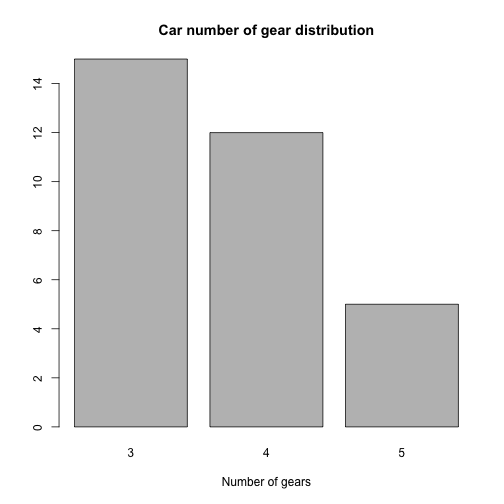You can make a horizontal bar plot.

barplot(counts, main = "Car distribution", horiz = T, names.arg = c("3 Gears",
"4 Gears", "5 Gears"))You can draw a grouped bar plot like this.

counts <- table(mtcars$cyl, mtcars$gear)
barplot(counts, main = "Gear and cylinder", xlab = "Number of gears", col = c("black",
"grey", "white"), legend = rownames(counts), beside = T)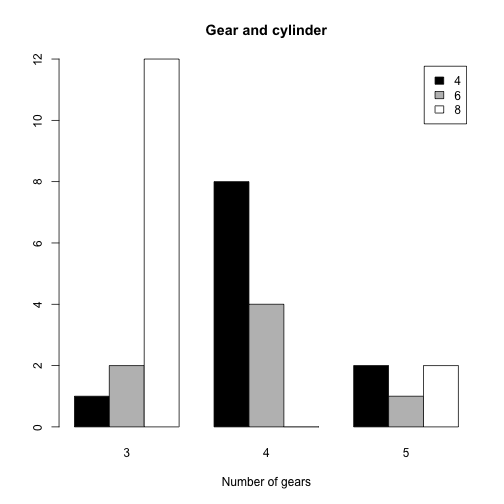## Barplots with error bars

There is no inbuilt way to generate error bars in R. But we have to hack a solution. We will use arrows to generate error bars. The trick is arrows take an argument of angle. If you give angle=90 it will generate an error bar.

## Generate some data
heights <- vector()  # Empty vector to hold the heights of the bar plot
stddevs <- vector()  # Empty vector to hold the standard deviations

for (i in 1:3) {
# We will generate 3 sets of data
d <- rnorm(10, mean = 5, sd = 1)  # Be sure that we have some positive data
heights[i] <- mean(d)
stddevs[i] <- sd(d)
}

## Draw the barplot
bp <- barplot(heights, names = c(1:3), ylim = c(0, 8), space = 2)  # Returns a matrix
mids <- bp[, 1]  # Get the midpoint

for (i in 1:3) {
# We will draw the errorbars
arrows(x0 = mids[i], y0 = heights[i] - stddevs[i], x1 = mids[i], y1 = heights[i] +
stddevs[i], code = 3, angle = 90)
}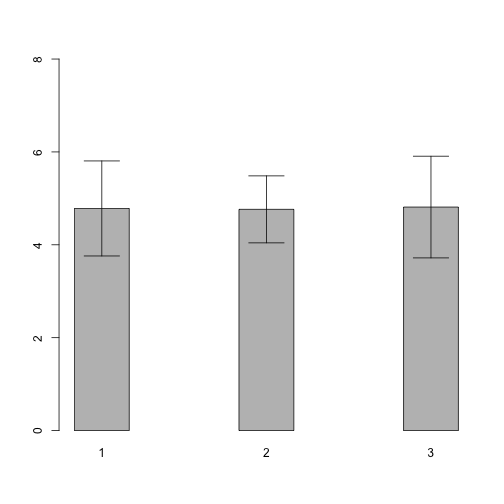# Colors in R

If you have drawn figures in Excel, you might have used colors like this

barplot(counts, main = "Gear and cylinder", xlab = "Number of gears", col = c("red",
"green", "blue"), legend = rownames(counts), beside = T)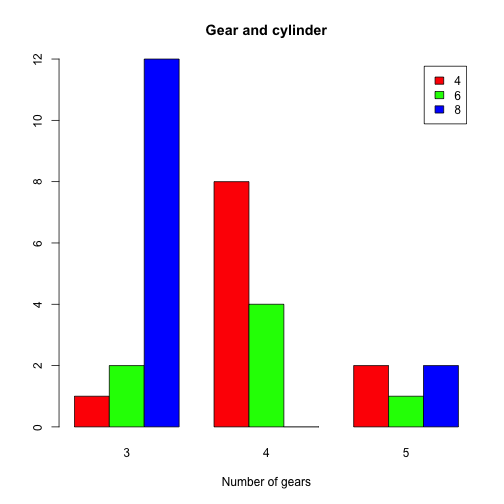This is what I call “Christmas” plot. You should never use color like this. In R you can use sophisticated color palette.

library(RColorBrewer)
## Warning: package 'RColorBrewer' was built under R version 3.0.2
display.brewer.all()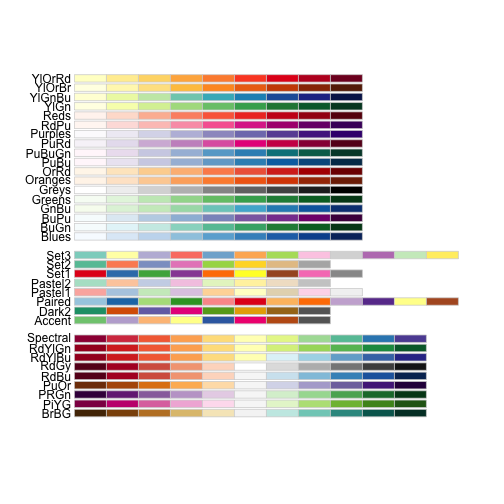There are 3 palettes: sequential, quantitative, and contrasting. Now choose a nice color for our plot.

nice <- brewer.pal(3, "Pastel2")
barplot(counts, main = "Gear and cylinder", xlab = "Number of gears", col = nice,
legend = rownames(counts), beside = T)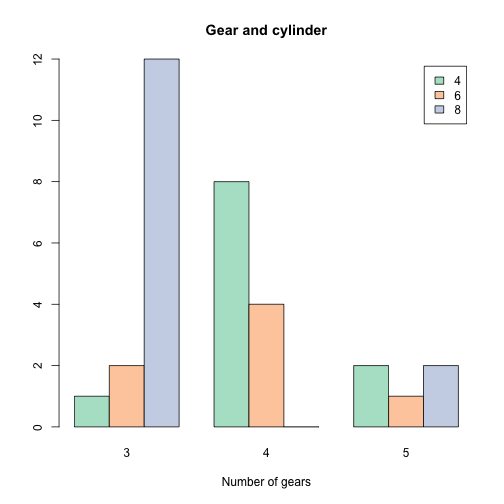# Linear regression and correlation

## Linear regression

We will use the women datasets in R for this example. These is a simple datasets with “weight” and “height” of women in the US.

data(women)
head(women)
##   height weight
## 1     58    115
## 2     59    117
## 3     60    120
## 4     61    123
## 5     62    126
## 6     63    129

If we plot a simple scatterplot of this data, we will see there is an obvious correlation between this two variables.

plot(women)Let’s do a linear regression between this two variables.

l <- lm(women$weight ~ women$height)
summary(l)
##
## Call:
## lm(formula = women$weight ~ women$height)
##
## Residuals:
##    Min     1Q Median     3Q    Max
## -1.733 -1.133 -0.383  0.742  3.117
##
## Coefficients:
##              Estimate Std. Error t value Pr(>|t|)
## (Intercept)  -87.5167     5.9369   -14.7  1.7e-09 ***
## women$height 3.4500 0.0911 37.9 1.1e-14 *** ## --- ## Signif. codes: 0 '***' 0.001 '**' 0.01 '*' 0.05 '.' 0.1 ' ' 1 ## ## Residual standard error: 1.53 on 13 degrees of freedom ## Multiple R-squared: 0.991, Adjusted R-squared: 0.99 ## F-statistic: 1.43e+03 on 1 and 13 DF, p-value: 1.09e-14 You can safely ignore the (Intercept) line. But the adjusted r2 is 0.99 with P-value 1.1e-14, which is highly significant. Let’s put the regression line on the plot. plot(women$height, women$weight, main = "Women height vs weight", xlab = "Height", ylab = "Weight") abline(l, lwd = 2, col = "red")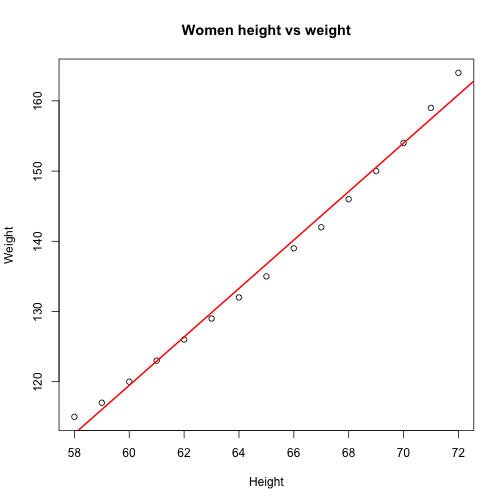You can extract the P-value and the r2 values for printing s <- summary(l) s$adj.r.squared
##  0.9903
s$coefficients[2, 4] ##  1.091e-14 ## Correlations We can also do a correlation between this two variables s <- cor.test(women$weight, women$height, alternatives = "tow.sided") s ## ## Pearson's product-moment correlation ## ## data: women$weight and women$height ## t = 37.86, df = 13, p-value = 1.088e-14 ## alternative hypothesis: true correlation is not equal to 0 ## 95 percent confidence interval: ## 0.9861 0.9985 ## sample estimates: ## cor ## 0.9955 s$p.value
##  1.088e-14
s\$estimate
##    cor
## 0.9955